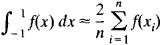# Chebyshev Formula

## Chebyshev Formula

a formula for the approximate computation of a definite integral:The formula is accurate for polynomials of degree not greater than n – 1, where n is the number of nodes. The values of the xi have been computed for some n. In the case of n = 9, for example, x1 = –x9 = 0.911589, x2 = –x8 = 0.601019, x3 = –x7 = 0.528762, x4 = –x6 = 0.167906, and x5 = 0. For n = 8 and n > 9, the xi have complex values; the Chebyshev formula can therefore be used only for n = 1, 2, 3, 4, 5, 6, 7, and 9. The for mula was derived by P. L. Chebyshev in 1873.

Site: Follow: Share:
Open / Close